output.to from Sideway
Fluid Mechanic

Fluid Kinematics

Draft for Information Only

Content

Fluid Kinematics
Velocity Field
Acceleration Field

Fluid Kinematics

Kinematics is the study of the velocity and acceleration of the fluid motion. There are two methods to study the motion of fluid. One way can focus on fluid particles by tracking their position vector, i.e.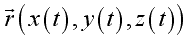and determining their associated properties as a function of time, i.e. velocity vector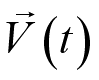. This is the Lagrangian description of fluid motion. The other way of describing fluid motion is the Eulerian description of fluid motion, which focuses on the fluid flows in and out of a control volume in terms of field variables. Instead of following individual fluid particles, the description describes the variables value of  the location only and does not care whichever fluid particle occupying that location inside the control volume  as a function of space and time, i.e.  pressure field, velocity field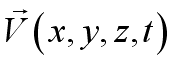to define the flow field.

Velocity Field

Fluid property can be represented as a function of spatial coordinates in term of a field representation. For a fluid flow, it is also a function of time.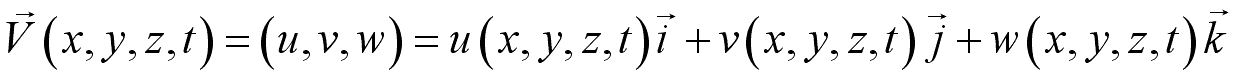When the velocity value of a location equals to zero,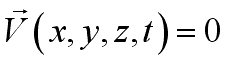i.e.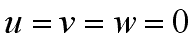, it is called a stagnation point.

Acceleration Field

In a fluid flow, the velocity of a particle is a function of its location and time. Imply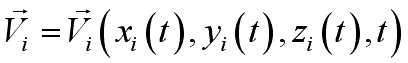. By definition, the acceleration of a particle is the time rate of change of its velocity,. Using chain rule of differentiation,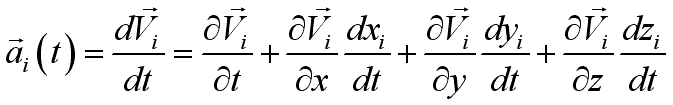and because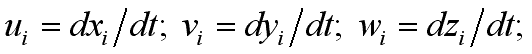imply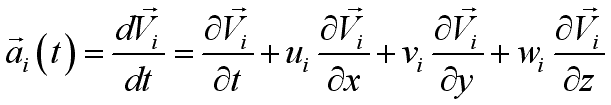.

Since the equation can be used to describe any particle, the acceleration field can be obtained from the velocity field as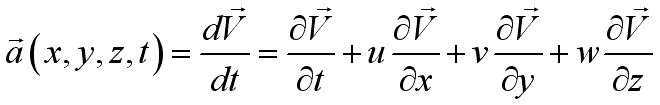,or;
where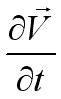is named local acceleration or unsteady acceleration, which equals to zero for steady flow and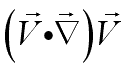is named advective acceleration or convective acceleration, which equals to non-zero even for steady flow.

And the total acceleration can be expressed as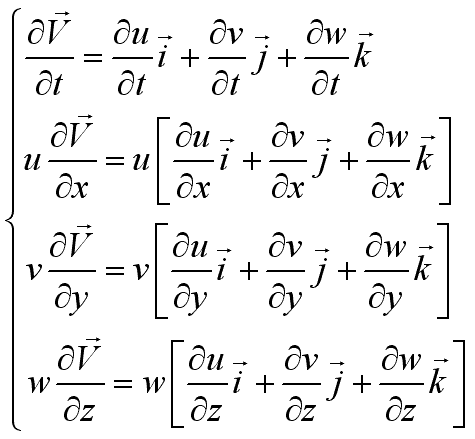or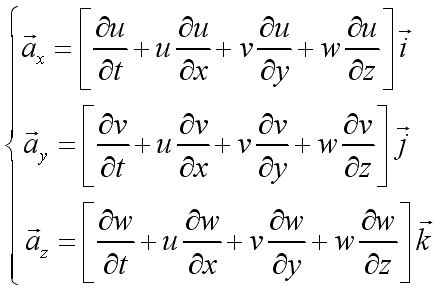Since the total derivative operator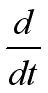is derived from a fluid particle, it is called material derivative,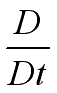. i.e.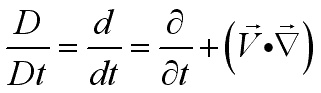The total acceleration is therefore also called material acceleration.

ID: 100100002 Last Updated: 2/7/2010 Revision: 1Home 5

Management

HBR 3

Information

Recreation

Culture

Chinese 1097

English 337

Computer

Hardware 160

Software

Application 205

Latex 35

Manim 203

Numeric 19

Programming

Web 285

Unicode 504

HTML 65

CSS 65

SVG 46ASP.NET 270

OS 422

Python 71Knowledge

Mathematics

Algebra 84

Geometry 32Calculus 67

Engineering

Mechanical

Rigid Bodies

Statics 92

Dynamics 37

Fluid Kinematics 5

Control

Natural Sciences

Electric 27